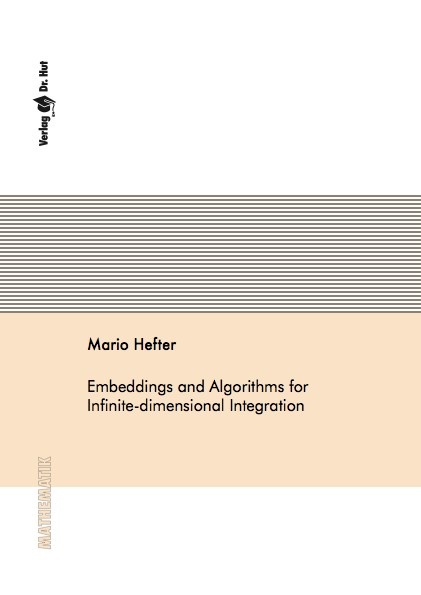Datenbestand vom 17. August 2019aktualisiert am 17. August 2019

# ISBN 9783843920988Euro 72,00 inkl. 7% MwSt

978-3-8439-2098-8, Reihe Mathematik

Mario Hefter
Embeddings and Algorithms for Infinite-dimensional Integration

131 Seiten, Dissertation Technische Universität Kaiserslautern (2014), Softcover, A5

## Zusammenfassung / Abstract

In this thesis we study the computational complexity of a particular instance of infinite-dimensional integration in the framework of information-based complexity. The task is to approximate the integral with respect to the standard Gaussian measure, which is given by the countable product of the standard normal distribution, on the space of real valued sequences. We assume that functions belong to a weighted reproducing kernel Hilbert space, which is associated to a family of weights. The value of a single weight controls how much a function from the unit ball of such a space is allowed to depend on the associated group of variables. The one-dimensional building block for the weighted reproducing kernel Hilbert space is a Sobolev space of once differentiable functions. Using the multi-level technique and results from tractability we construct an algorithm for this problem and show its optimality.

A motivation for studying infinite-dimensional integration is as follows. Functions with infinitely many variables naturally occur in the context of quadrature problems of stochastic differential equations. This is due to the fact that typically the basic probabilistic objects, e.g., the driving Brownian motion, are naturally represented via a random series of functions.

We also study embeddings between weighted reproducing kernel Hilbert spaces.